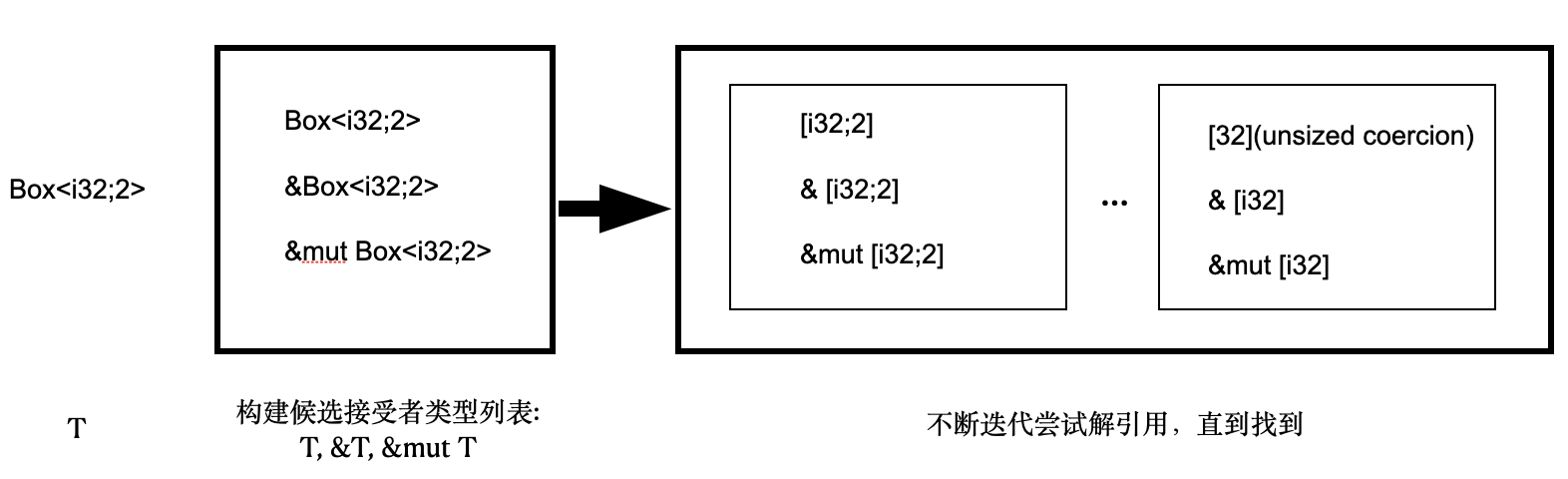# 引子

``````
struct Thing {
field: String
}

fn f1(sth: &Thing)  {
let tmp = *sth;
//            ┗━ Move data out of `thing`.

}``````

``````
//doesn't compile.
fn f1(thing: &Thing) {
let tmp = *thing;
//          ┃ ┗━ Point directly to the referenced data.
//          ┗━━━ Try to copy RHS's value, otherwise move it into `tmp`.
}

//Compiles.
fn f2(sth: &Thing) -> &String {
&(*sth).field
}

fn main() {
let x = Thing {field: String::from("hello")};
f1(&x);
}``````

• 参考资料

# 那么在Rust中什么时候会产生Move?

• 该值必须是引用语义，即分配到Heap上的类型(如String,Vec,包含String等引用语义成员的Struct，Box<T>中的T等)；否则，值语义的类型对变量值重新绑定的时候执行的是复制，而非所有权转移.
• 需要有新的变量，以重新绑定

• 值语义:按位复制以后，与原始对象无关。
• 引用语义:也叫指针语义。一般是指将数据存储于堆内存中，通过栈内存的指针 来管理堆内存的数据，并且引用语义禁止按位复制。按位复制就是指栈复制，也叫浅复制，它只复制栈上的数据。相对而言，深复制就是对栈上和堆上的数据一起复制。

1. 赋值语句`=`
``````
let s = String::from("hello");
let x = s;  //Move``````
1. 作为函数参数或函数返回值

``````
// Cannot Compile
fn test(x:String){}

fn main() {
let s = "hello".to_string();
test(s);   //Move
println!("{}",s);
}``````
1. for,while和loop循环语句

因为for循环实际上是一个语法糖，rust编译器会将`for val in v`替换成`for val in IntoIterator::into_iter(v)`,此时转换为第2种作为函数参数的场景

``````
let v = vec![1,2,3];
for val in v{   //Move
println!("{}",val);
}
println!("{:?}", v);``````

1. {}词法作用域 在新的词法作用域中，Rust会重新声明变量，导致发生所有权转移
``````
let outer_sp = "hello".to_string();
{
outer_sp;  //Move
}
println!("{}", outer_sp);  ``````
1. if let和 while let语句，重新绑定
``````
let a = Some("hello".to_string());
if let Some(s) = a {  //Move
println!("{:?}",a);
}``````
1. match的词法作用域
``````
let a = Some("hello".to_string());
match a {
_ => println!("test")
}
println!("{:?}", a);
match a {//-------------------------------------------
Some(s) => println!("{}", s),  //Move    |
_ => println!("test")                          //|match scope
} //--------------------------------------------------
println!("{:?}", a);``````

• `match a`本身不会转移a的所有权，而是在作用域中对a内部的值进行绑定为s时，才会Move。
• println!语句只需要获取a的不可变引用`& a`即可，因此也不会转移a的所有权。

``````
enum BTree {
Leaf(i32),
Node(Box&lt;BTree>, i32, Box&lt;BTree>)
}

fn sampleTree() -> BTree{
let l1 = Box::new(BTree::Leaf(1));
let l2 = Box::new(BTree::Leaf(3));
let n1 = Box::new(BTree::Node(l1,2,l2));
let r2 = Box::new(BTree::Leaf(5));
BTree::Node(n1, 4, r2)
}

fn tree_weight(t: &BTree) -> i32 {
match *t{
BTree::Node(ref l, payload,ref r )=> {
}
}
}

fn main() {
let t = sampleTree();
assert_eq!(tree_weight(&t), 1+2+3+4+5);
}``````
1. 闭包

``````
let a = "hello".to_string();
let c = || {a;};  //Move
// print!("{}", a);
c();
c();   //由于a被Move到闭包中，因此c为FnOnce，不能再次执行``````

## 小Quiz:

``````
#[derive(Debug)]
enum Color {
Red,
Blue
}

impl Color {
fn to_str(&self) -> &str {
match *self {
Color::Red => "red",
Color::Blue => "blue"
}
}
}

fn main() {
let c = Color::Red;
c.to_str();
println!("{:?}",c);
}``````

# 理解引用的其他常见误区

1. 对于实现了`Deref Trait`的类型x，x.deref()的类型与*x一致吗?

``````
impl ops::Deref for String {
type Target = str;

#[inline]
fn deref(&self) -> &str {
unsafe { str::from_utf8_unchecked(&self.vec) }
}
}``````

``````
s: String
&s: &String
x.deref(): &str
*x: str
&*s: &str``````
1. 方法调用的时候只进行Deref吗？ 实际上除了Deref外，还会进行事先进行一次构建引用列表的操作，整个过程如下:• 1.方法接收者类型为T
• 2.构建方法接收者候选类型列表: T, &T，&mut T
• 3.对上述三种类型分别进行解引用得到:*T, T, mut T
• 4.循环上述过程直至到unsized coercion转换